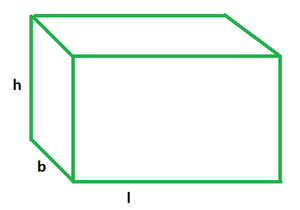Open in App
Not now

# Volume of a Rectangular Prism Formula

• Last Updated : 16 Sep, 2022

Such a polygon that has two parallel bases is called a prism. So, a rectangular prism is one whose two parallel bases are rectangular. Apart from those two, it has four rectangular faces as well. It is a three-dimensional geometric figure with two congruent and parallel bases and belongs to the polyhedron family. It has six rectangular faces, twelve edges, and eight vertices. Books, geometry boxes, cereal boxes, containers, rooms, cabinets, mobiles, fish tanks, etc., are some examples of rectangular prisms that we see in our daily lives. There are two kinds of rectangular prisms, namely, the right rectangular prism and the oblique, rectangular prism. A rectangular prism can be regarded as a cuboid. It is shown as follows:## Volume of a Rectangular Prism

The quantity of tri space contained in a surface is expressed as a scalar quantity called volume. For example, the amount of space occupied or contained by material or 3D objects. The SI-derived unit, the cubic meter, is frequently used to quantify volume numerically.

The entire space inside a rectangular prism is measured by the volume of the prism. Consider a water-filled rectangular container. In this situation, the box’s capacity is equal to the entire amount of water it can store.

The formula for the volume of a rectangular prism is equal to the product of its base area and its height.

The volume of a Rectangular Prism (V) = Base Area × Height of the Prism

Formula

V = l × b × h

Where l, b, and h are the length, breadth, and height of the rectangular prism respectively.

## Solved Examples on Volume of a Rectangular Prism

Example 1: Find the volume of a rectangular prism whose length, breadth, and height are 12, 15, and 8 cm.

Solution:

Given: l = 12 cm

b = 15 cm

h = 8 cm

Volume = l × b × h

= 12 × 15 × 8

= 1440 cm3

Example 2: Find the base area of a rectangular prism whose volume is 40 cm3 and height is 4 cm.

Solution:

Given: V = 40 cm3

h = 4 cm

Since, V = l × b × h or,

V = Base Area × Height

40 cm3 = Base Area × 4 cm

⇒ Base Area = 40/4

= 10 cm2

Example 3: Find the volume of a rectangular prism if its base area is 50 cm2 and height is 12 cm.

Solution:

Given: Base area = l × b = 50 cm2

h = 12 cm

Volume = l × b × h

= 50 × 12

= 600 cm3

Example 4: Find the height of a rectangular prism given its volume is 600 cm3 and the base area is 50 cm2.

Solution:

Given: V = 600 cm3

Base area = l × b = 50 cm2

Volume = l × b × h

600 = 50 × h

h = 600/50

= 12 cm

Example 5: Determine the volume of a rectangular prism if its height is 15 inches and the length and breadth of its base are 11 inches and 6 inches, respectively.

Solution:

Given data,

l = 11 inches

b = 6 inches

h = 15 inches

We know that,

The volume of a Rectangular Prism = (l × b × h) cubic units

= 12 × 11 × 6 = 792 cubic inches

Hence, the volume of the given prism is 792 cu. in.

Example 6: Find the volume of a rectangular prism whose length, breadth, and height are 10, 9, and 8 cm.

Solution:

Given: l = 10 cm

b = 9 cm

h = 8 cm

Volume = l × b × h

= 10 × 9 × 8

= 720 cm3

Example 7: Determine the volume of a rectangular prism if its height is 10 cm and the length and breadth of its base are 8 cm and 5 cm, respectively.

Solution:

Given data,

l = 8 cm

b = 5 cm

h = 10 cm

We know that,

The volume of a Rectangular Prism = (l × b × h) cubic units

= 10 × 8 × 5 = 400 cu. cm

Hence, the volume of the given prism is 400 cu. cm.

My Personal Notes arrow_drop_up